GRE Subject Test: Math : Trigonometric Integrals

Example Questions

Example Question #9 : Integrals

Integrate the following.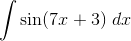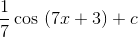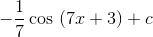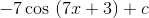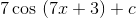Explanation:

We can integrate the function by using substitution where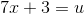so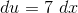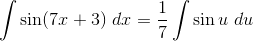Just focus on integrating sine now: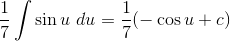The last step is to reinsert the substitution:Example Question #10 : Integrals

Integrate the following.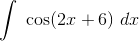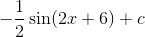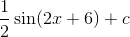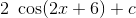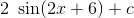Explanation:

We can integrate using substitution: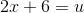and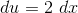so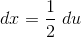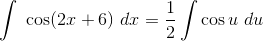Now we can just focus on integrating cosine: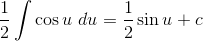Once the integration is complete, we can reinsert our substitution:Example Question #1 : Trigonometric Integrals

Evaluate the following integral.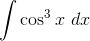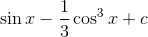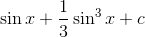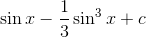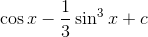Explanation:

Recall: The identity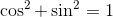The integral can be rewritten as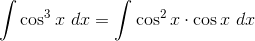Because of the trig identity above, we can rewrite it in a different way: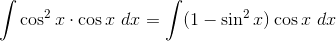Now we can integrate using substitution where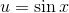and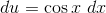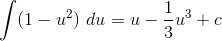Finally, we reinsert our substitution:Example Question #2 : Trigonometric Integrals

Evaluate the following integral.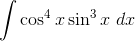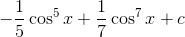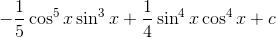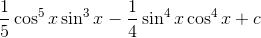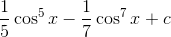Explanation:

Recall: The trig identity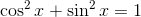We can rewrite the integral using the above identity as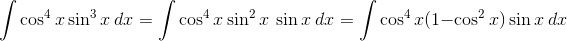We can now solve the integral using substitution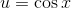and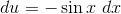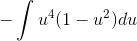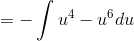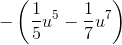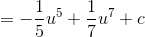The last step is to reinsert our substitution:Example Question #51 : Derivatives & Integrals

Fnd the derivative of tan(x) with respect to x or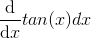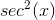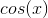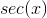Derivative cannot be found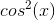Explanation:

The is one of the trigonometric integrals that must be memorized.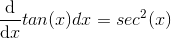Other common trig derivatives that should be memorized are: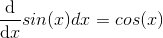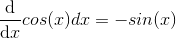Example Question #1 : Trigonometric Integrals

Evaluate: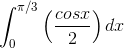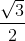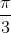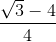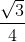Explanation:

1) The 1/2 is a constant, and so is pulled out front.

2) The integral of cos(x) is sin(x), by definition.

3) Writing the limits for evaluation: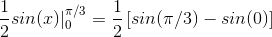4) Using the unit circle,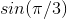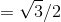, and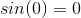.

5)Simplifying: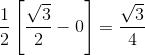All GRE Subject Test: Math Resources